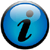# Filtering input

Perl's text processing features are ideally suited for filtering operations that extract the desired content from a file.
1. Skip empty lines and lines containing only white space:
```perl -lne 'print if (/\S/)' input > output

```
2. Filter on absolute fold-changes and p-values, located in this case in columns 3 and 5 (indexed 2 and 4), respectively:
```perl -lane 'print if (abs(\$F) >= 2 and \$F <= 0.05)' input > output

```
3. Extract lines that contain Ensembl gene identifiers:
```perl -lne 'print if (/ENSG\d+/)' input > output

```
4. Extract lines that contain membrane-related terms, case-insensitive:
```perl -lne 'print if (/membrane/i)' input > output

```
5. Sub-sampling -- extract approximately 1 percent of random lines from an input file:
```perl -lne '\$i = rand; print if (\$i <= 0.01)' input > output
```